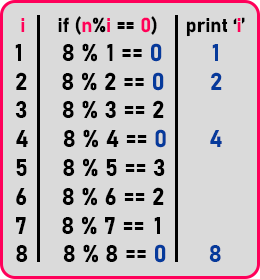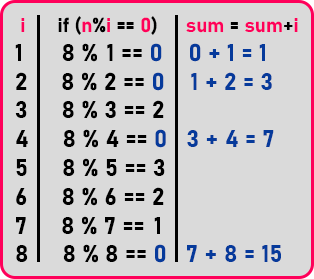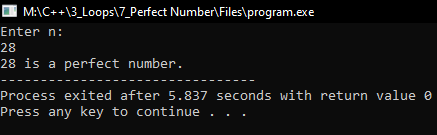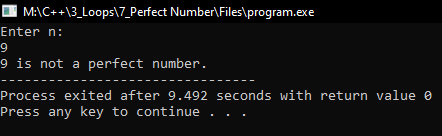# Perfect Number using Loop in C++

## Perfect Number using Loop in C++

In this article, I am going to discuss the Program to Check Perfect Number using Loop in C++ with Examples. Please read our previous articles, where we discussed Factors of a Number using Loop in C++ with Examples. Here, we will write a program for checking whether the number is a perfect number or not.

##### Perfect Number:

A perfect number is the sum of factors of the given number that is equal to twice the number. For example, factors of ‘6’ are ‘1’, ‘2’, ‘3’ and ‘6’. So, the sum of its factors is ‘1 + 2 + 3 + 6 = 12’, that is ‘12’ and twice the number that is ‘6 * 2 = 12’. Both are the same so ‘6’ is a perfect number.Above is the same example which we used in the previous article. If the sum of the factor is double the number, then it is known as the perfect number. To check a number is a perfect number or not, let us first calculate the sum of factors. For adding factors, we are taking a variable of the name ‘sum’ and initializing the ‘sum’ variable with ‘0’.Here we modified the 3rd column. Instead of printing the value, we are modifying the value of the sum as we found the factor of the given number. So, you can see this in the table. We have a detailed explanation of the above table in the previous article of ‘Factor of a Number’. You can see the explanation there.

So, this was the procedure to calculate the sum of the factors. So, we have calculated the sum of the factors, now we have to just check whether the sum is equal to double the number or not. Let’s see the program to understand this more clearly.

##### Program to check number is perfect number or not using loop in C++:
```#include <iostream>
using namespace std;
int main()
{
int n, sum = 0;
cout << "Enter n:" << endl;
cin >> n;

for (int i = 1; i <= n; i++)
{
if (n % i == 0)
{
sum = sum + i;
}
}
if (2 * n == sum)
cout << n << " is a perfect number.";
else
cout << n << " is not a perfect number.";
return 0;
}
```
###### Output1:###### Output2: